# Linear and Absolute Value Functions Linear Functions Are

• Slides: 30Linear and Absolute Value Functions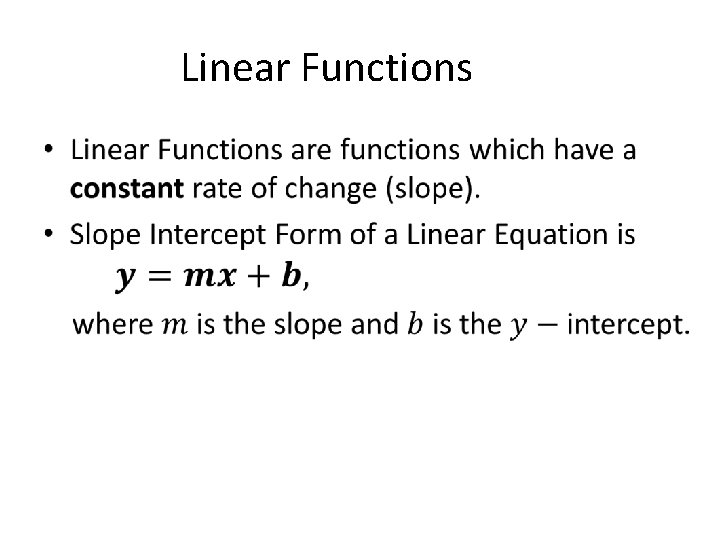Linear Functions •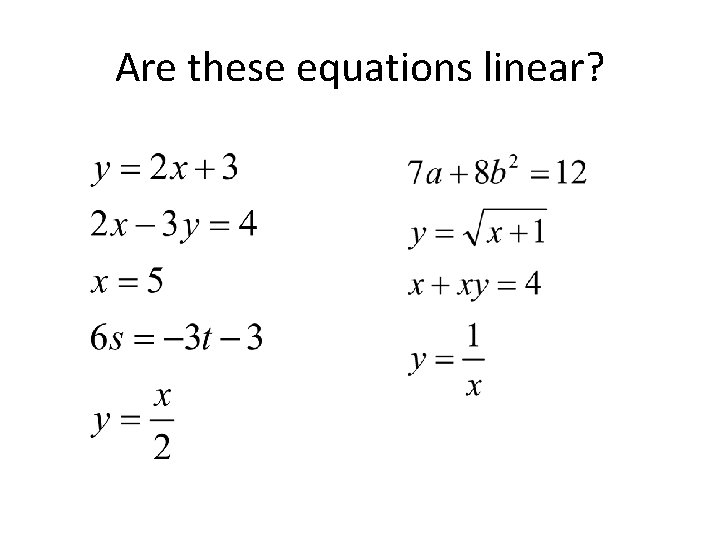Are these equations linear?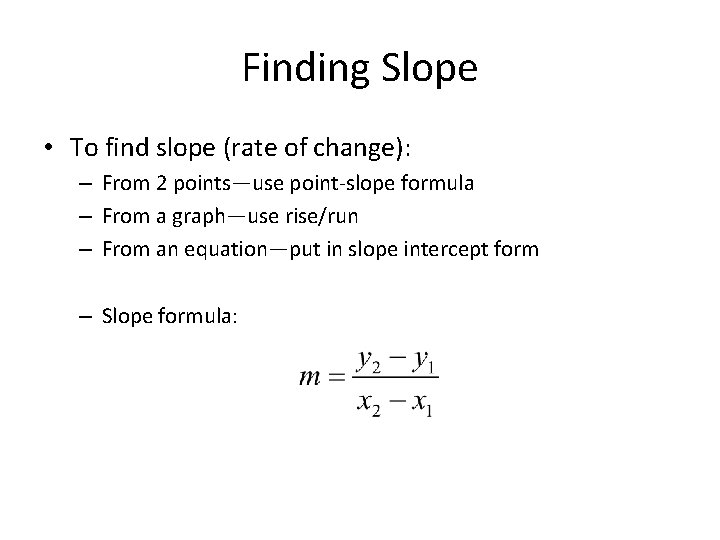Finding Slope • To find slope (rate of change): – From 2 points—use point-slope formula – From a graph—use rise/run – From an equation—put in slope intercept form – Slope formula: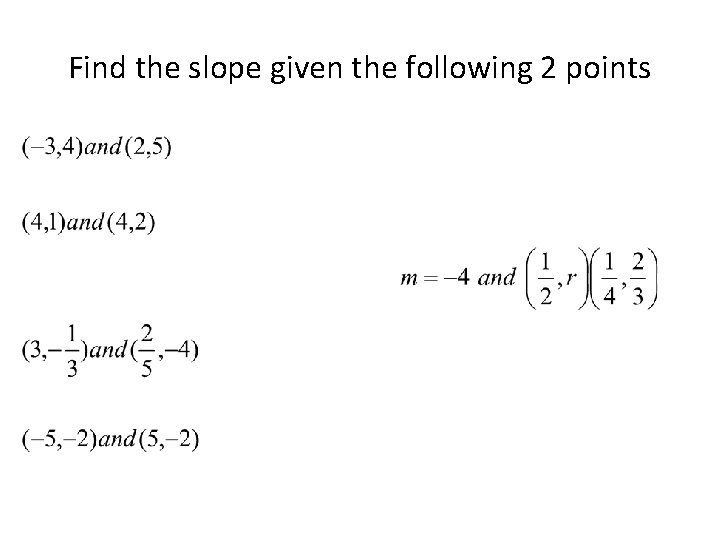Find the slope given the following 2 points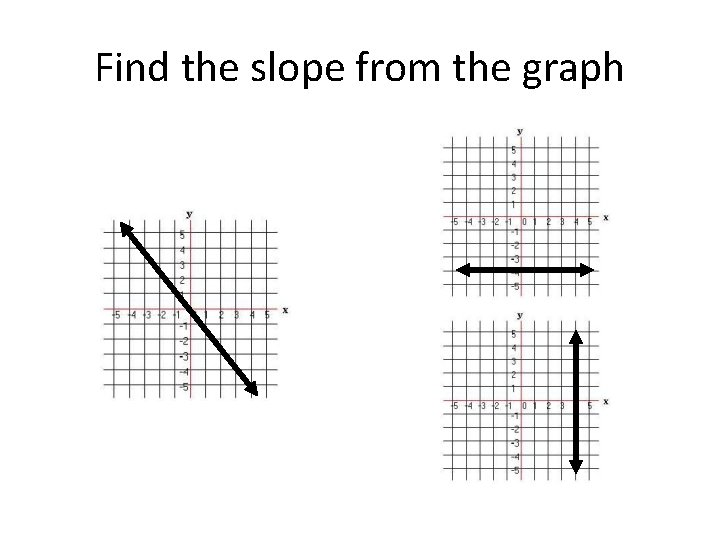Find the slope from the graphDo these ordered pairs represent linear functions? •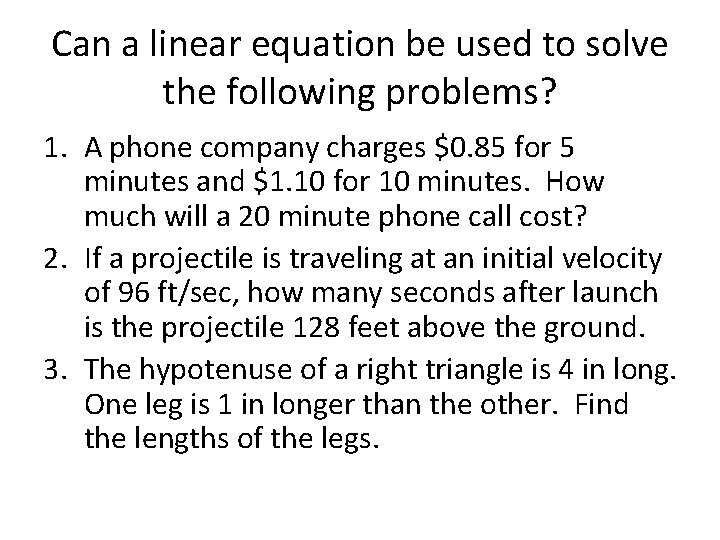Can a linear equation be used to solve the following problems? 1. A phone company charges \$0. 85 for 5 minutes and \$1. 10 for 10 minutes. How much will a 20 minute phone call cost? 2. If a projectile is traveling at an initial velocity of 96 ft/sec, how many seconds after launch is the projectile 128 feet above the ground. 3. The hypotenuse of a right triangle is 4 in long. One leg is 1 in longer than the other. Find the lengths of the legs.Writing Equations of Lines • To write the equation of a line, you must know a point and the slope • Use the point slope formula to write the equation • Put the final equation in slope intercept form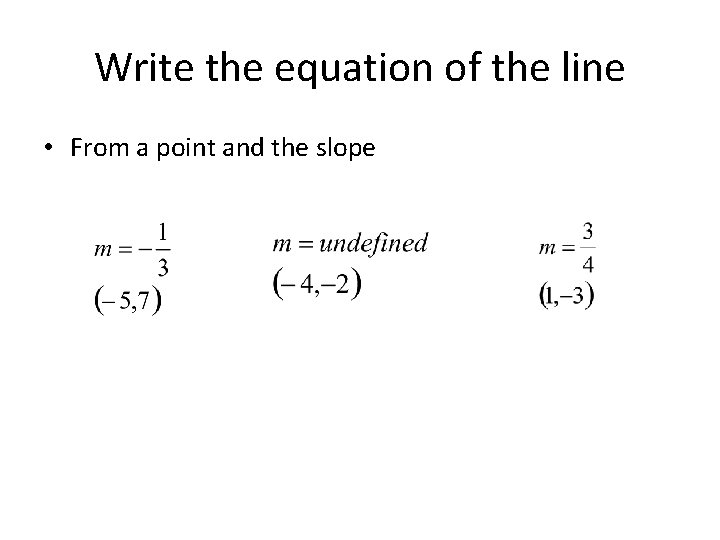Write the equation of the line • From a point and the slope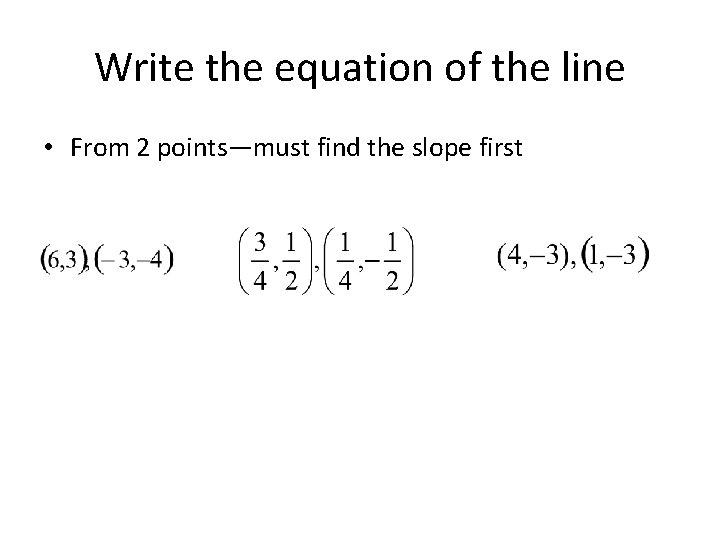Write the equation of the line • From 2 points—must find the slope first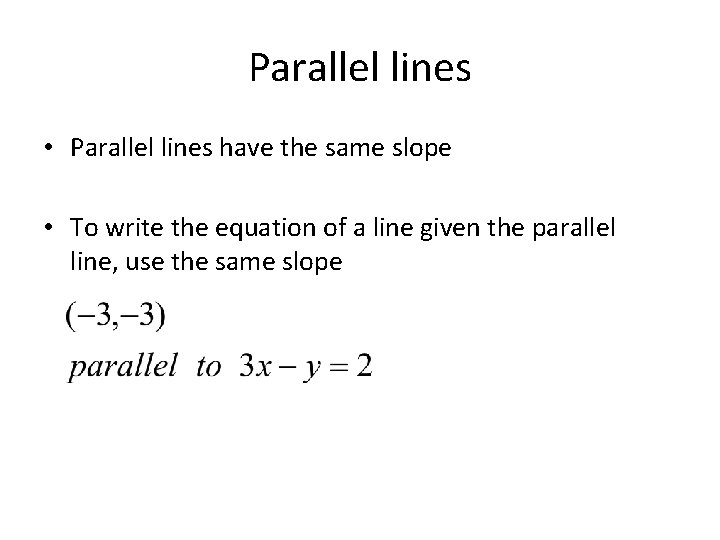Parallel lines • Parallel lines have the same slope • To write the equation of a line given the parallel line, use the same slopeParallel lines • To write the equation of a line given the parallel line, use the same slopePerpendicular lines • Perpendicular lines have opposite reciprocal slopes • To write the equation of a line given the perpendicular line, flip the slope and change the sign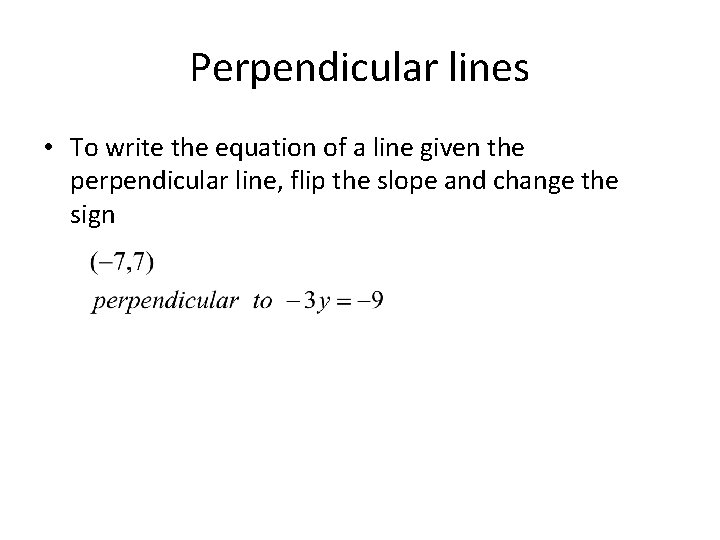Perpendicular lines • To write the equation of a line given the perpendicular line, flip the slope and change the sign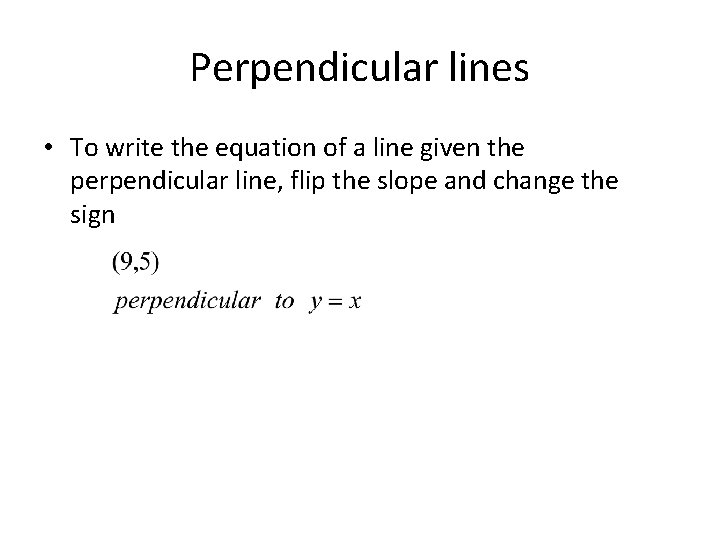Perpendicular lines • To write the equation of a line given the perpendicular line, flip the slope and change the sign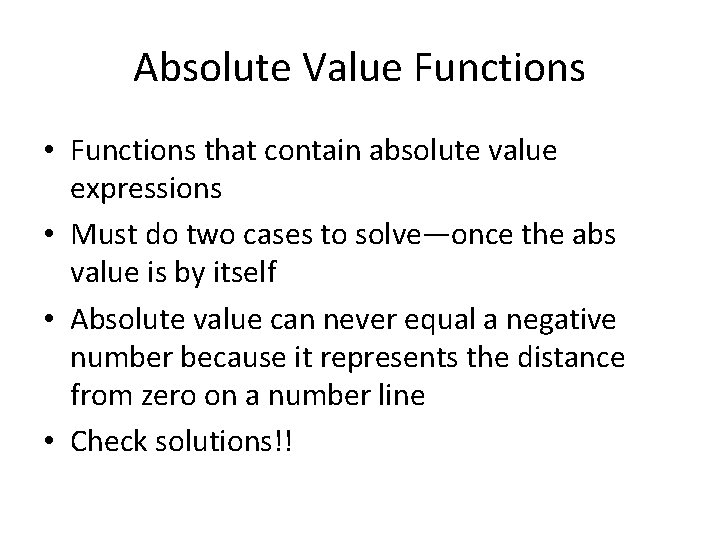Absolute Value Functions • Functions that contain absolute value expressions • Must do two cases to solve—once the abs value is by itself • Absolute value can never equal a negative number because it represents the distance from zero on a number line • Check solutions!!Solve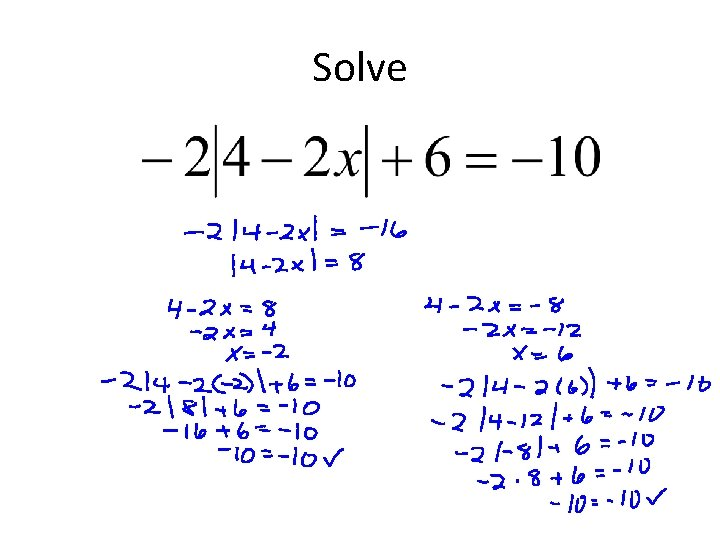Solve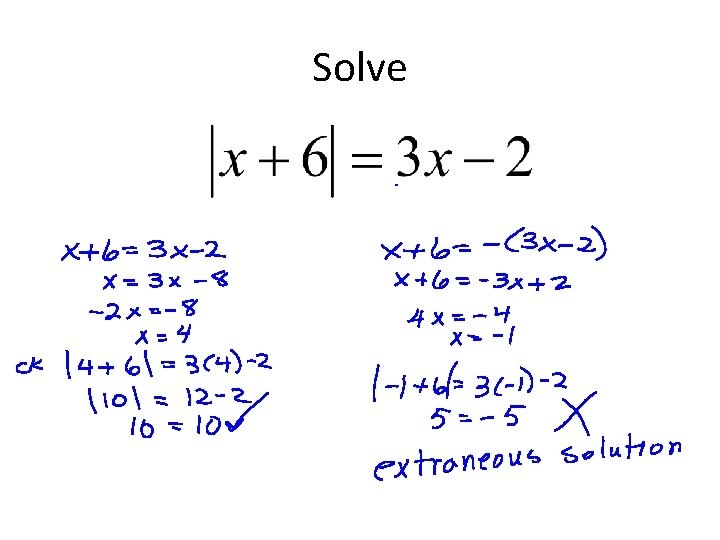Solve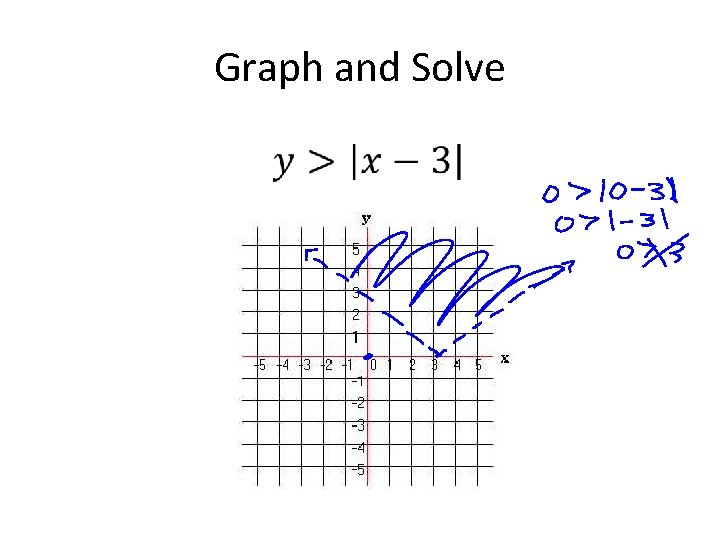Graph and SolveGraph and Solve •Graph and Solve •Graph and SolveAbsolute Value Inequalities • Inequalities containing an absolute value expression • Solve just like an absolute value equation • Just remember < “and” > “or” • Special cases when < or > a negative #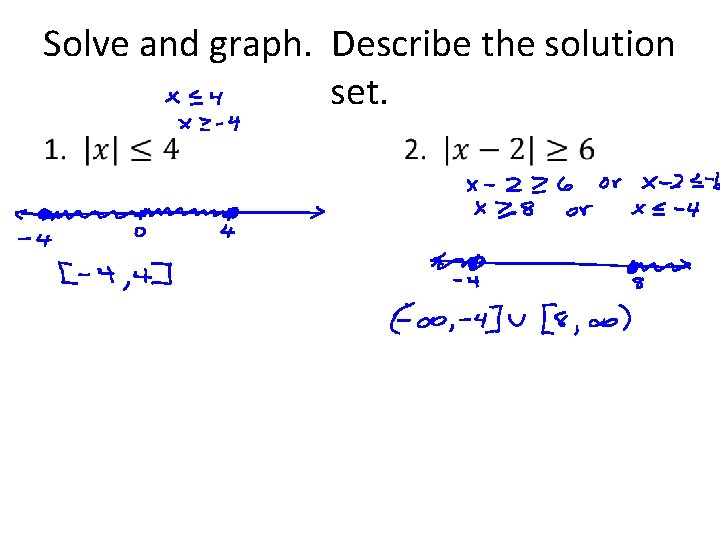Solve and graph. Describe the solution set. •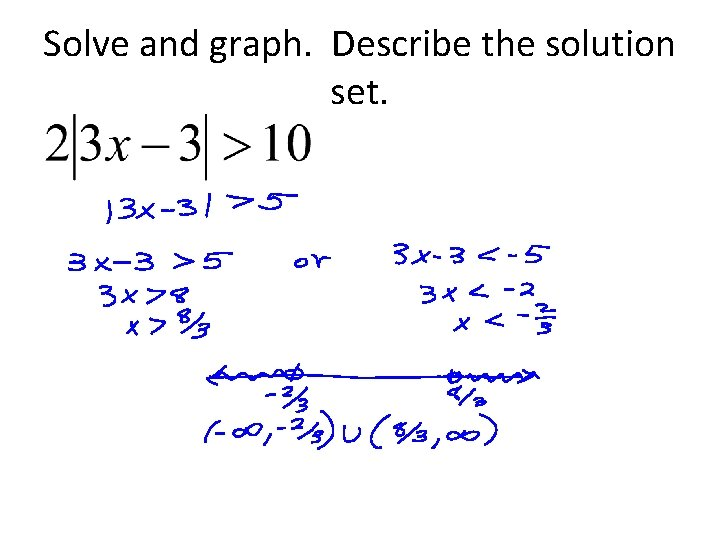Solve and graph. Describe the solution set.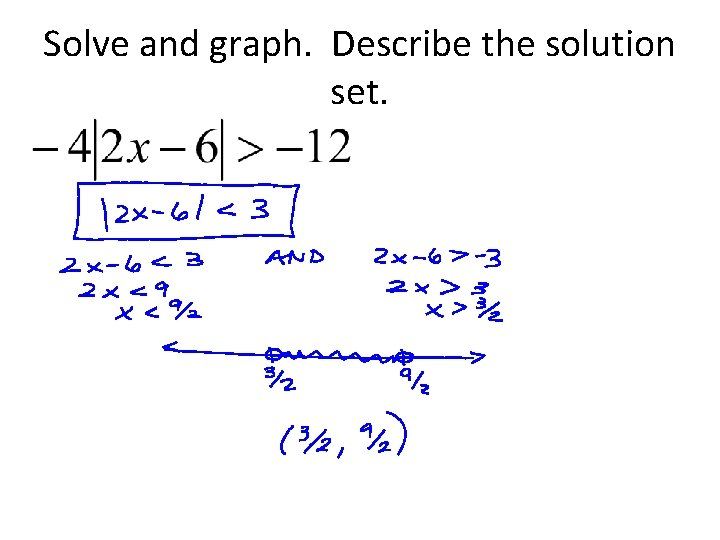Solve and graph. Describe the solution set.Solve and graph. Describe the solution set.Solve and graph. Describe solution sets.